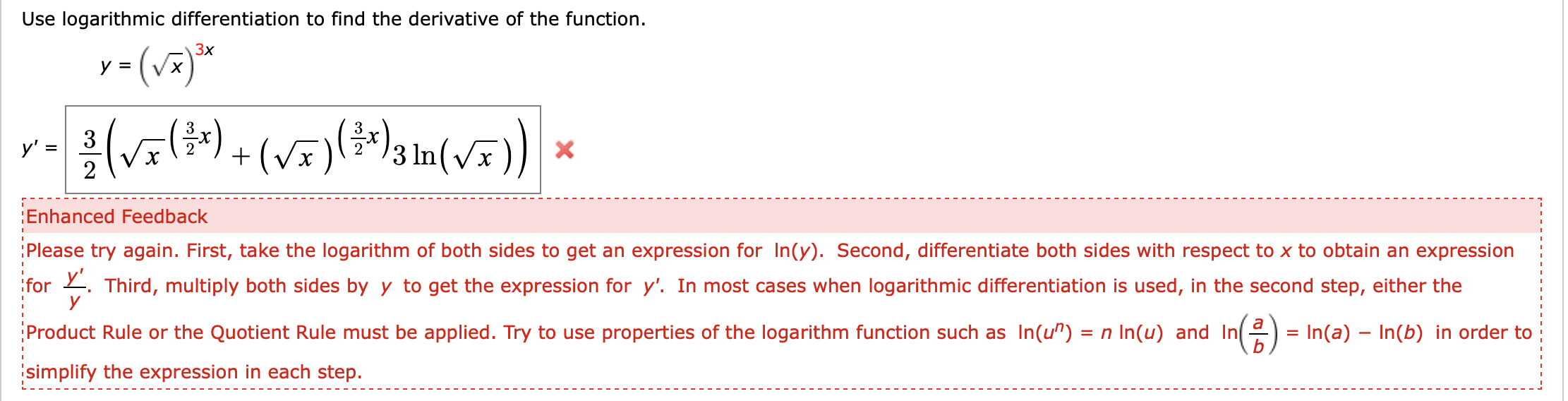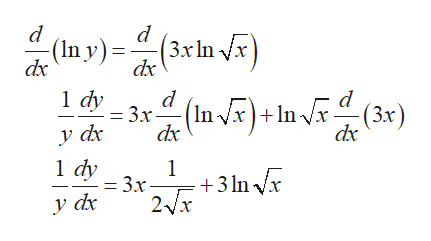# Use logarithmic differentiation to find the derivative of the functiony()3xy =)3y' =2Enhanced FeedbackPlease try again. First, take the logarithm of both sides to get an expression for In(y). Second, differentiate both sides with respect to x to obtain an expressionfor Third, multiply both sides byyto get the expression for y'. In most cases when logarithmic differentiation is used, in the second step, either theaProduct Rule or the Quotient Rule must be applied. Try to use properties of the logarithm function such as In(u") = n In(u) and InIn(a)- In(b) in order tosimplify the expression in each step.

Question
3 viewshelp_outlineImage TranscriptioncloseUse logarithmic differentiation to find the derivative of the function y() 3x y = ) 3 y' = 2 Enhanced Feedback Please try again. First, take the logarithm of both sides to get an expression for In(y). Second, differentiate both sides with respect to x to obtain an expression for Third, multiply both sides by y to get the expression for y'. In most cases when logarithmic differentiation is used, in the second step, either the a Product Rule or the Quotient Rule must be applied. Try to use properties of the logarithm function such as In(u") = n In(u) and In In(a)- In(b) in order to simplify the expression in each step. fullscreen
check_circle

Step 1

Take log on both sides and differentiate with respect to x,

Step 2

Differentiate using th...help_outlineImage Transcriptionclosed (In y) = dx (3xln x dx 1 dy = 3x dx nvx)+lnJx^^(3x) y dr 1 dy 1 = 3x +3 In Vr 2x y d fullscreen

### Want to see the full answer?

See Solution

#### Want to see this answer and more?

Solutions are written by subject experts who are available 24/7. Questions are typically answered within 1 hour.*

See Solution
*Response times may vary by subject and question.
Tagged in

### Derivative